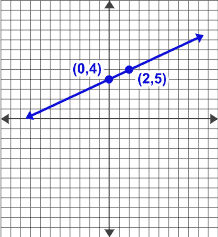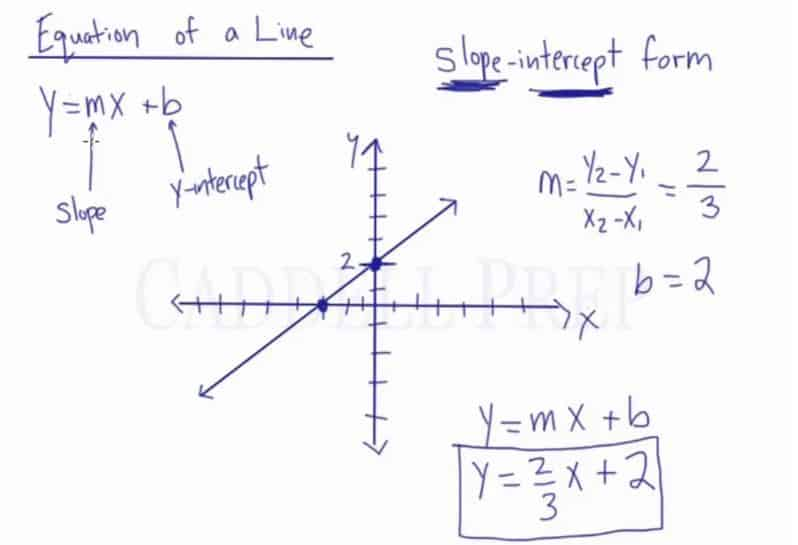Learn about the equation of a line. After you finish this lesson, view all of our Pre-Algebra lessons and practice problems.

## Equation of a Line (Slope-Intercept Form)$y=mx+b$

where m = slope and b = y-intercept.

To find the slope, use the slope formula:$m = \dfrac{y_2 - y_1}{x_2 - x_1}$

## Examples of Equation of a Line

### Example 1First, let’s determine the coordinates of the points$P_1(-1,3)$ and$P_2(0,-3)$

Then, solve for the slope$m = \dfrac{y_2 - y_1}{x_2 - x_1}$$m = \dfrac{-3-3}{0-{-}1}$$m=-6$

Slope-intercept form is$y = mx + b$

Where$m$ = slope and$b$ =$y$-intercept

Now, our y-interecept is$-3$$b = -3$

Substitute the given and we have$y = -6x-3$

### Example 2

First, let’s determine the coordinates of the points$P_1(0,4)$ and$P_2(2,5)$

Then, solve for the slope$m = \dfrac{y_2 - y_1}{x_2 - x_1}$$m = \dfrac{5-4}{2-0}$$m=\dfrac{1}{2}$

Slope-intercept form is$y = mx + b$

Where$m$ = slope and$b$ =$y$-intercept

Now, our y-interecept is$4$$b = 4$

Substitute the given and we have$y = \dfrac{1}{2}x+4$

## Video-Lesson Transcript

In this lesson, we’ll review the equation of a line and go over the slope-intercept form which is the most common equation of a line.

It’s called slope-intercept form for a very specific reason.

When we look at the equation, we will know what our slope is and its intercept.

Intercept is where a line segment intersects the axis. The$y$-intercept is the point where the line segment crosses or touches the$y$-axis.

Slope-intercept form is$y = mx + b$

Where$m =$ slope and$b = y$-intercept

Our formula for slope is$m = \dfrac{y_1 - y_2}{x_1 - x_2}$

Let’s look at the graph.

Then count the change in$y$ and$x$ from the origin with$(0, 0)$coordinates.

So we have$m = \dfrac{2}{3}$

Now, let’s refer back to the graph and look where the line intersects the$y$-axis. Meaning, find the point where the line meets the$y$– axis and where the$x$-axis is$0$.

Now, our$y$-interecept is$2$.

So now$b = 2$.

Now, we’re ready to write the slope-intercept form.

Substitute the given and we have$y = mx + b$$y = \dfrac{2}{3} x + 2$

So our slope-interecept form is$y = \dfrac{2}{3} x + 2$Again, the slope-intercept form is$y = mx + b$

Where$m =$ slope and$b = y$-intercept.